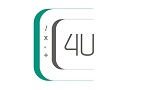# Rhombus Area and Perimeter Calculator

Rhombus Area Calculator tool is used to calculate area and perimeter of a rhombus.## What is a Rhombus ?

A quadrilateral, all sides are equal in length, and opposite sides are parallel. The attributes indicate that a rhombus can also be classified as a parallelogram and all parallelograms are also classified as a trapezoid. The diagonals of a rhombus bisect one another but because they bisect each other at 90° angles, we call these diagonals perpendicular bisectors.

Area=b x h

Perimeter=4b

#### Properties of Rhombus

• Opposite sides are congruent (they equal each other).
• Opposite angles are congruent (they equal each other).
• Consecutive angles are supplementary (they add up to 180).
• Diagonals bisect each other (the parts are equal).
• Diagonals perpendicular (the form right angles in the middle).
• Diagonals bisect angles (the angles are equal to each other).
• All four sides are congruent.
• The diagonals are NOT congruent.